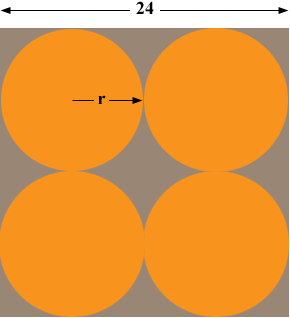SEARCH HOMEMath Central Quandaries & QueriesQuestion from Daniela, a student: four circles are drawn in a square such that the circles are tangent to each other as shown. find the area of the shaded region. It the goes on to show a diagram with a square and four circles drawn in it. The length of a side of the square is 24. Please help me!Hi Daniela,

Is this the diagram?What is the area of the square?

What is the radius r of the circles?

What is the area of a circle r?

What is the area remaining when you remove 4 circles of radius r from the square?

PennyMath Central is supported by the University of Regina and The Pacific Institute for the Mathematical Sciences.